﻿ 基于神经网络的土壤含水量高光谱估测

# 基于神经网络的土壤含水量高光谱估测Hyper Spectral Estimation of Soil Water Content Based on Neural Network

Abstract: Hyperspectral remote sensing is widely used in rock minerals, soil, vegetation and other fields due to its extremely high spectral resolution. Using the spectral reflectance data of 84 soil samples in a certain place, by analyzing the relationship between reflectance spectrum and soil water content, the BP (back propagation) neural network inversion model was established by mathematically transforming the spectral data and using the inversion factors obtained by the correlation analysis method. The results show that in the prediction of soil water content, the model established by BP neural network is effective, and the first-order differential time model with spectral transformation to square root has higher precision.

1. 引言

2. 土壤含水量BP神经网络估测模型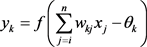(1)

BP神经网络计算模型如下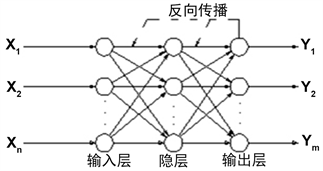Figure 1. BP network structure diagram

$y={f}^{2}\left({\theta }^{2}{f}^{1}\left({\theta }^{1}x+{b}^{1}\right)+{b}^{2}\right)$ (2)

3. 算例

3.1. 光谱数据的平滑处理

$\begin{array}{l}{R}_{i}=0.04{{R}^{\prime }}_{i-4}+0.08{{R}^{\prime }}_{i-3}+0.12{{R}^{\prime }}_{i-2}+0.16{{R}^{\prime }}_{i-1}+0.20{{R}^{\prime }}_{i}+0.16{{R}^{\prime }}_{i+1}\\ \text{}+0.12{{R}^{\prime }}_{i+2}+0.08{{R}^{\prime }}_{i+3}+0.04{{R}^{\prime }}_{i+4}\end{array}$ (4)

3.2. 光谱数据变换方法Table 1. Transformation method table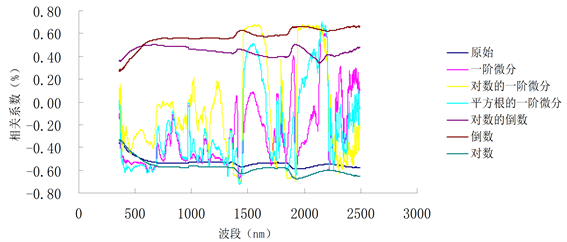Figure 2. Transformation comprehensive map

3.3. 数据处理过程

4. 结果分析Table 2. Accuracy comparison between first-order differential models of square rootsTable 3. Accuracy comparison between first-order differential modelsTable 4. Accuracy comparison between first-order differential models of logarithms

5. 结束语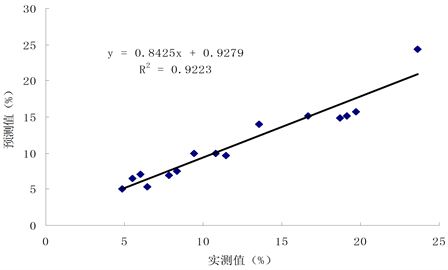Figure 3. BP neural network test sample accuracy analysis chart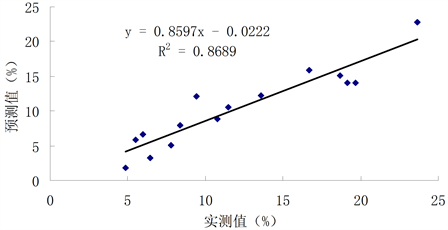Figure 4. Multiple linear regression test samples accuracy analysis chartTable 5. BP neural network test sample accuracy analysis tableTable 6. Linear regression test sample accuracy analysis table

 尚璇, 李西灿, 徐邮邮, 刘莎莎. 土壤水与有机质对高光谱的作用及交互作用规律[J]. 中国农业科学, 2017(8): 1465-1475.

 谢伯承, 薛绪掌, 刘伟东, 等. 基于包络线法对土壤光谱特征的提取及其分析[J]. 土壤学报, 2005, 42(1): 171-175.

 郑曼迪, 熊黑钢, 乔娟峰, 等. 基于高光谱的不同人类干扰程度下荒漠土壤有机质含量估算模型[J]. 干旱区地理, 2018, 41(2): 384-392.

 丁国香. 基于神经网络的土壤有机质及全铁含量的高光谱反演研究[D]: [硕士学位论文]. 南京: 南京信息工程大学, 2008.

 程朋根, 吴剑. 土壤有机质高光谱遥感和地统计定量预测[J]. 农业工程学报, 2009, 25(3): 142-147.

 何挺, 王静. 土壤有机质光谱特征研究[J]. 武汉大学学报·信息科学版, 2006, l1(31): 975-979.

 贺军亮, 蒋建军. 土壤有机质含量的高光谱特性及其反演[J]. 中国农业科学, 2007, 40(3): 638-643.

 唐启义, 冯明光. DPS数据处理系统–实验设计统计分析及数据挖掘[M]. 北京: 科学出版社, 2007: 625-676.

 He, J.L., Jiang, J.J., Zhou, S.L., et al. (2007) The Hyperspectral Characteristics and Retrieval of Soil Organic Matter Content. Scientia Agricultura Sinica.

 戴昌达. 中国主要土壤光谱反射特性分类与数据处理的初步研究[M]. 遥感文集. 北京: 北京科学出版社, 1981: 315-323.

 卢艳丽, 白由路. 基于主成分回归分析的土壤有机质高光谱预测与模型验证[J]. 植物营养与肥料学报, 2008, 14(6): 1076-1082.

Top